# Problem in finding a general solution

• navneet9431
So, options (A) and (B) are correct, as well as (C) and (D). In summary, the conversation discusses solving a trigonometric equation using the double-angle formulas and the given options for the solution are (A), (B), (C), and (D). The correct solutions are (A) and (B), and also (C) and (D).

Gold Member

## Homework Statement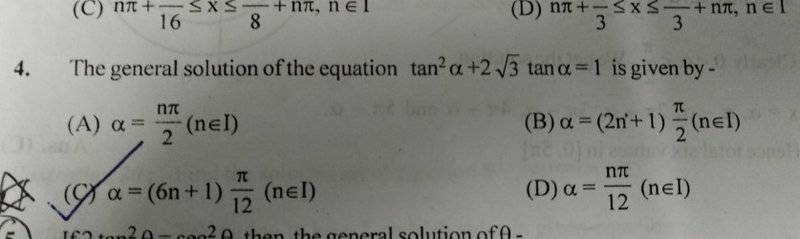## Homework Equations

General Formula for Tan(a)=Tan(b)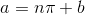## The Attempt at a Solution

See the question I have uploaded.

I have tried solving it this way,

Firstly I applied the Quadratic Formula to get,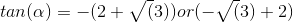Now we have two cases,

CASE-1

When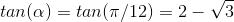So General Formula here will be,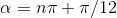Now, CASE-2

when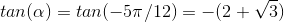So General Formula here will be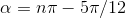I do not know what should I do next to get the answer? Please tell me how to proceed Further.

The answer given in the key is the option (C).

I will be thankful for any help!

#### Attachments

Hello,

So you need to combine ##\pi (n+ {1\over 12}) ## and ##\pi (n - {5\over 12}) ## . Leave the ##\pi## outside the brackets and try a few n. The pattern emerges !

•scottdave
if the 6n looks scary in the option C, it is actually just ##n/2## cause ##a=(6n+1)\frac{\pi}{12}=\frac{n}{2}\pi+\frac{\pi}{12}##. From this very last expression for a, what do you get if you put
1) n=even=2k
2) n=odd=2k+1

•ehild and scottdave
I think the problem is solved by @Delta2's hint, so I may come with a very simple solution of the equation tan2(α)+2√3 tan(α)=1,which can be rearranged to 1-tan2(α)=2√3 tan(α).
nπ/2 in the offered solutions suggests to solve the equation for 2α. The double-angle formula is ##\tan(2α)=\frac{2\tan(α)}{1-\tan^2(α)}##, that is ##\tan(2α)=\frac{2\tan(α)}{2\sqrt3 \tan(α)}=\frac{1}{\sqrt 3}##, that is, 2α=π/6+kπ and α=π/12+kπ/2.

•Delta2